# Navier-Stokes Forward Simulation¶

Now let's target a somewhat more complex example: a fluid simulation based on the Navier-Stokes equations. This is still very simple with ΦFlow (phiflow), as differentiable operators for all steps exist there. The Navier-Stokes equations (in their incompressible form) introduce an additional pressure field $p$, and a constraint for conservation of mass, as introduced in equation {eq}model-boussinesq2d. We're also moving a marker field, denoted by $d$ here, with the flow. It indicates regions of higher temperature, and exerts a force via a buouyancy factor $\xi$:

\begin{aligned} \frac{\partial \mathbf{u}}{\partial{t}} + \mathbf{u} \cdot \nabla \mathbf{u} &= - \frac{1}{\rho} \nabla p + \nu \nabla\cdot \nabla \mathbf{u} + (0,1)^T \xi d \quad \text{s.t.} \quad \nabla \cdot \mathbf{u} = 0, \\ \frac{\partial d}{\partial{t}} + \mathbf{u} \cdot \nabla d &= 0 \end{aligned}

Here, we're aiming for an incompressible flow (i.e., $\rho = \text{const}$), and use a simple buoyancy model (the Boussinesq approximation) via the term $(0,1)^T \xi d$. This models changes in density without explicitly calculating $\rho$, and we assume a gravity force that acts along the y direction via the vector $(0,1)^T$. We'll solve this PDE on a closed domain with Dirichlet boundary conditions $\mathbf{u}=0$ for the velocity, and Neumann boundaries $\frac{\partial p}{\partial x}=0$ for pressure, on a domain $\Omega$ with a physical size of $100 \times 80$ units. [run in colab]

## Implementation¶

As in the previous section, the first command with a "!" prefix installs the phiflow python package from GitHub via pip in your python environment. (Skip or modify this command if necessary.)

In :
#!pip install --upgrade --quiet phiflow
!pip install --upgrade --quiet git+https://github.com/tum-pbs/[email protected]

from phi.flow import *  # The Dash GUI is not supported on Google colab, ignore the warning
import pylab


## Setting up the simulation¶

The following code sets up a few constants, which are denoted by upper case names. We'll use $40\times32$ cells to discretize our domain, introduce a slight viscosity via $\nu$, and define the time step to be $\Delta t=1.5$.

We're creating a first CenteredGrid here, which is initialized by a Sphere geometry object. This will represent the inflow region INFLOW where hot smoke is generated.

In :
DT = 1.5
NU = 0.01

INFLOW = CenteredGrid(Sphere(center=(30,15), radius=10), extrapolation.BOUNDARY, x=32, y=40, bounds=Box[0:80, 0:100]) * 0.2


The inflow will be used to inject smoke into a second centered grid smoke that represents the marker field $d$ from above. Note that we've defined a Box of size $100x80$ above. This is the physical scale in terms of spatial units in our simulation, i.e., a velocity of magnitude $1$ will move the smoke density by 1 unit per 1 time unit, which may be larger or smaller than a cell in the discretized grid, depending on the settings for x,y. You could parametrize your simulation grid to directly resemble real-world units, or keep appropriate conversion factors in mind.

The inflow sphere above is already using the "world" coordinates: it is located at $x=30$ along the first axis, and $y=15$ (within the $100x80$ domain box).

Next, we create grids for the quantities we want to simulate. For this example, we require a velocity field and a smoke density field.

In :
smoke = CenteredGrid(0, extrapolation.BOUNDARY, x=32, y=40, bounds=Box[0:80, 0:100])  # sampled at cell centers
velocity = StaggeredGrid(0, extrapolation.ZERO, x=32, y=40, bounds=Box[0:80, 0:100])  # sampled in staggered form at face centers


We sample the smoke field at the cell centers and the velocity in staggered form. The staggered grid internally contains 2 centered grids with different dimensions, and can be converted into centered grids (or simply numpy arrays) via the unstack function, as explained in the link above.

Next we define the update step of the simulation, which calls the necessary functions to advance the state of our fluid system by dt. The next cell computes one such step, and plots the marker density after one simulation frame.

In :
def step(velocity, smoke, pressure, dt=1.0, buoyancy_factor=1.0):
smoke = advect.semi_lagrangian(smoke, velocity, dt) + INFLOW
buoyancy_force = smoke * (0, buoyancy_factor) >> velocity  # resamples smoke to velocity sample points
velocity = advect.semi_lagrangian(velocity, velocity, dt) + dt * buoyancy_force
velocity = diffuse.explicit(velocity, NU, dt)
velocity, pressure = fluid.make_incompressible(velocity)
return velocity, smoke, pressure

velocity, smoke, pressure = step(velocity, smoke, None, dt=DT)

print("Max. velocity and mean marker density: " + format( [ math.max(velocity.values) , math.mean(smoke.values) ] ))

pylab.imshow(np.asarray(smoke.values.numpy('y,x')), origin='lower', cmap='magma')

Max. velocity and mean marker density: [0.15530995, 0.008125]

Out:
<matplotlib.image.AxesImage at 0x7fa2f8d16eb0>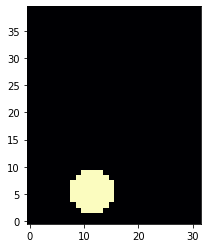A lot has happened in this step() call: we've advected the smoke field, added an upwards force via a Boussinesq model, advected the velocity field, and finally made it divergence free via a pressure solve.

The Boussinesq model uses a multiplication by a tuple (0, buoyancy_factor) to turn the smoke field into a staggered, 2 component force field, sampled at the locations of the velocity components via the >> operator. This operator makes sure the individual force components are correctly interpolated for the velocity components of the staggered velocity. >> could be rewritten via the sampling function at() as (smoke*(0,buoyancy_factor)).at(velocity). However, >> also directly ensure the boundary conditions of the original grid are kept. Hence, it internally also does StaggeredGrid(..., extrapolation.ZERO,...) for the force grid. Long story short: the >> operator does the same thing, and typically produces shorter and more readable code.

The pressure projection step in make_incompressible is typically the computationally most expensive step in the sequence above. It solves a Poisson equation for the boundary conditions of the domain, and updates the velocity field with the gradient of the computed pressure.

Just for testing, we've also printed the mean value of the velocities, and the max density after the update. As you can see in the resulting image, we have a first round region of smoke, with a slight upwards motion (which does not show here yet).

## Datatypes and dimensions¶

The variables we created for the fields of the simulation here are instances of the class Grid. Like tensors, grids also have the shape attribute which lists all batch, spatial and channel dimensions. Shapes in phiflow store not only the sizes of the dimensions but also their names and types.

In :
print(f"Smoke: {smoke.shape}")
print(f"Velocity: {velocity.shape}")
print(f"Inflow: {INFLOW.shape}, spatial only: {INFLOW.shape.spatial}")

Smoke: (xˢ=32, yˢ=40)
Velocity: (xˢ=32, yˢ=40, vectorᵛ=2)
Inflow: (xˢ=32, yˢ=40), spatial only: (xˢ=32, yˢ=40)


Note that the phiflow output here indicates the type of a dimension, e.g., $^S$ for a spatial, and $^V$ for a vector dimension. Later on for learning, we'll also introduce batch dimensions.

The actual content of a shape object can be obtained via .sizes, or alternatively we can query the size of a specific dimension dim via .get_size('dim'). Here are two examples:

In :
print(f"Shape content: {velocity.shape.sizes}")
print(f"Vector dimension: {velocity.shape.get_size('vector')}")

Shape content: (32, 40, 2)
Vector dimension: 2


The grid values can be accessed using the values property. This is an important difference to a phiflow tensor object, which does not have values, as illustrated in the code example below.

In :
print("Statistics of the different simulation grids:")
print(smoke.values)
print(velocity.values)

# in contrast to a simple tensor:
test_tensor = math.tensor(numpy.zeros([2, 5, 3]), spatial('x,y'), channel('vector'))
print("Reordered test tensor shape: " + format(test_tensor.numpy('vector,y,x').shape) )
#print(test_tensor.values.numpy('y,x')) # error! tensors don't return their content via ".values"

Statistics of the different simulation grids:
(xˢ=32, yˢ=40) float32  0.0 < ... < 0.20000000298023224
(xˢ=(31, 32), yˢ=(40, 39), vectorᵛ=2) float32  -0.12352858483791351 < ... < 0.15530994534492493
Reordered test tensor shape: (3, 5, 2)


Grids have many more properties which are documented here. Also note that the staggered grid has a non-uniform shape because the number of faces is not equal to the number of cells (in this example the x component has $31 \times 40$ cells, while y has $32 \times 39$). The INFLOW grid naturally has the same dimensions as the smoke grid.

## Time evolution¶

With this setup, we can easily advance the simulation forward in time a bit more by repeatedly calling the step function.

In :
for time_step in range(10):
velocity, smoke, pressure = step(velocity, smoke, pressure, dt=DT)
print('Computed frame {}, max velocity {}'.format(time_step , np.asarray(math.max(velocity.values)) ))

Computed frame 0, max velocity 0.4609803557395935
Computed frame 1, max velocity 0.8926814794540405
Computed frame 2, max velocity 1.4052708148956299
Computed frame 3, max velocity 2.036500930786133
Computed frame 4, max velocity 2.921010971069336
Computed frame 5, max velocity 3.828195810317993
Computed frame 6, max velocity 4.516851425170898
Computed frame 7, max velocity 4.861286640167236
Computed frame 8, max velocity 5.125314235687256
Computed frame 9, max velocity 5.476282119750977


Now the hot plume is starting to rise:

In :
pylab.imshow(smoke.values.numpy('y,x'), origin='lower', cmap='magma')

Out:
<matplotlib.image.AxesImage at 0x7fa2f902cf40>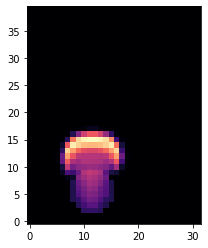Let's compute and show a few more steps of the simulation. Because of the inflow being located off-center to the left (with x position 30), the plume will curve towards the right when it hits the top wall of the domain.

In :
steps = [[ smoke.values, velocity.values.vector, velocity.values.vector ]]
for time_step in range(20):
if time_step<3 or time_step%10==0:
print('Computing time step %d' % time_step)
velocity, smoke, pressure = step(velocity, smoke, pressure, dt=DT)
if time_step%5==0:
steps.append( [smoke.values, velocity.values.vector, velocity.values.vector] )

fig, axes = pylab.subplots(1, len(steps), figsize=(16, 5))
for i in range(len(steps)):
axes[i].imshow(steps[i].numpy('y,x'), origin='lower', cmap='magma')
axes[i].set_title(f"d at t={i*5}")

Computing time step 0
Computing time step 1
Computing time step 2
Computing time step 10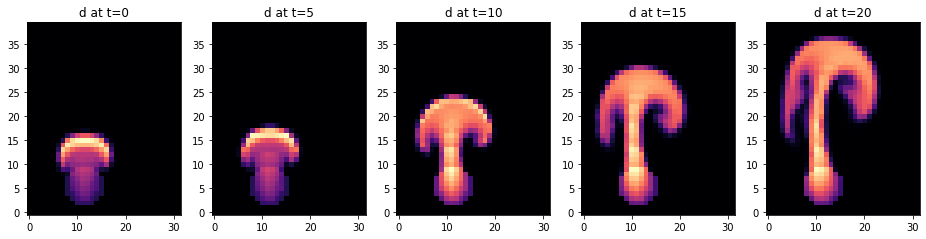We can also take a look at the velocities. The steps list above already stores vector and vector components of the velocities as numpy arrays, which we can show next.

In :
fig, axes = pylab.subplots(1, len(steps), figsize=(16, 5))
for i in range(len(steps)):
axes[i].imshow(steps[i].numpy('y,x'), origin='lower', cmap='magma')
axes[i].set_title(f"u_x at t={i*5}")

fig, axes = pylab.subplots(1, len(steps), figsize=(16, 5))
for i in range(len(steps)):
axes[i].imshow(steps[i].numpy('y,x'), origin='lower', cmap='magma')
axes[i].set_title(f"u_y at t={i*5}")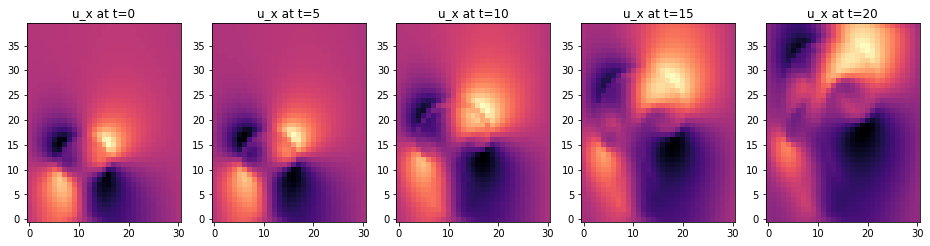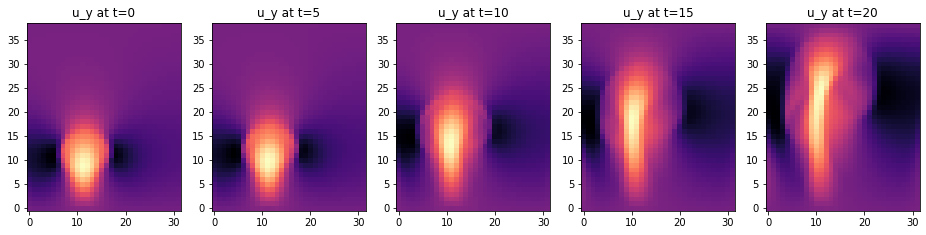It looks simple here, but this simulation setup is a powerful tool. The simulation could easily be extended to more complex cases or 3D, and it is already fully compatible with backpropagation pipelines of deep learning frameworks.

In the next chapters we'll show how to use these simulations for training NNs, and how to steer and modify them via trained NNs. This will illustrate how much we can improve the training process by having a solver in the loop, especially when the solver is differentiable. Before moving to these more complex training processes, we will cover a simpler supervised approach in the next chapter. This is very fundamental: even when aiming for advanced physics-based learning setups, a working supervised training is always the first step.

## Next steps¶

You could create a variety of nice fluid simulations based on this setup. E.g., try changing the spatial resolution, the buoyancy factors, and the overall length of the simulation run.